# Immanant

In connection with his work on the representation theory of groups (cf. also Schur functions in algebraic combinatorics), I. Schur introduced the following class of matrix functions. For a characterof a subgroupof the symmetric group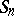(cf. also Symmetric group; Character of a group), the associated generalized matrix function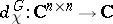acts on the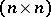-matrix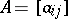byWhenand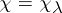is an irreducible character ofindexed by the partitionof, D.E. Littlewood [a25], Chap. VI, called the matrix function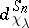an immanant. He used the immanants of certain matrices whose entries are symmetric functions to define the Schur functions (cf. also Schur functions in algebraic combinatorics). The immanantis also denoted by the less cumbersome notation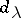.

The familiar determinant and permanent functions of a matrix are examples of immanants, and they correspond to the immanants associated with the alternating, respectively the trivial, character of. Indeed, for a matrix,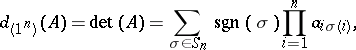where the signatureifis an even permutation andotherwise (cf. also Permutation), andGiven the plethora of inequalities and identities that involve the determinant and permanent functions, it is natural to seek generalizations of these relations to other immanants. For instance, in 1918 Schur [a40] obtained the following spectacular generalization of the Hadamard–Fischer inequality for positive semi-definite Hermitian matrices.

Schur's inequality: Letbe a character of the subgroupofand let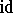be the identity permutation. For a positive semi-definite Hermitian matrixone has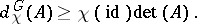(a1)

If one defines the normalized immanant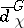to be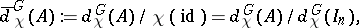where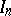is the identity-matrix, then Schur's inequality (a1) may be written as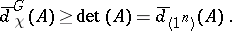The permanental analogue of the Hadamard inequality for the determinant of a positive semi-definite Hermitian matrix was obtained by M. Marcus [a26] in 1963, when he showed that for any positive semi-definite Hermitian matrix,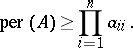In 1966, Marcus' inequality was generalized by E.H. Lieb [a24], who showed that for a positive semi-definite Hermitian matrixthat is partitioned in the form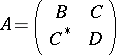, where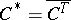denotes the transpose conjugate of, one has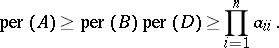In the same paper [a24], the following permanental analogue of Schur's inequality was conjectured.

The permanental dominance conjecture, or permanent-on-top conjecture (POT conjecture), states that for all positive semi-definite Hermitian matrices,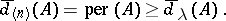(a2)

More generally, given two irreducible characters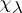and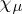of, one writes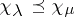iffor all positive semi-definite Hermitian matrices. This gives a partial order on the characters and also the immanants. In this context, Schur's inequality says that the alternating character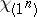is the smallest element of the resulting partially ordered set and the permanent-on-top conjecture asserts that the trivial character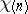is the largest element. A clear exposition on this topic can be found in [a33], Chap. 7.

There was little work done in this area before the appearance of a series of papers [a13], [a19], [a20], [a22], [a28] by several authors in the mid 1980s. These papers provide a partial resolution to the conjectured inequality in (a2), by showing that the permanent dominates the immanants associated with various characters. Since then there has been much progress; see, e.g., [a14], [a15], [a23], [a31], [a41]; and especially [a17], [a18], [a21], [a34], [a35], [a36], [a37], [a38], [a39], for the nature of the partial order on the characters. For instance, P. Heyfron [a17] proved that the hook immanants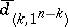are ordered as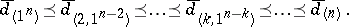(a3)

The ordering in (a3) is part of a more general result of Th.H. Pate [a34] saying that if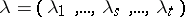is a non-increasing partition ofwith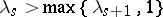and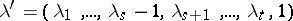, then. The POT conjecture has been established for all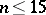with the exception of the three partitions,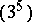and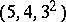by Pate in [a39]. The article [a39] gives a good survey of the results related to the POT conjecture and also discusses some topics related to immanants. In [a41], G.W. Soules considered an alternative approach to the POT conjecture through the eigenvalues of the Schur power matrix. In a similar vein, R. Merris [a31] investigated the extent to which the POT conjecture depends on the theory of group representations.

Immanants of other families of matrices besides the positive semi-definite Hermitian matrices have been studied. In [a11] I.P. Goulden and D.M. Jackson studied immanants of Jacobi–Trudi matrices and incidence matrices of directed trees. Their work inspired further results and conjectures about immanants of Jacobi–Trudi matrices and totally positive matrices, see, e.g., [a12], [a16], [a42], [a44].

Immanants of combinatorial matrices, such as the Laplacian matrix of graphs, have been considered in [a2], [a5], [a7], [a32]. Given a graphonvertices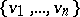, the Laplacian matrixofis the-matrix whose diagonal element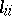is the degree of the vertex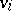and for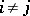,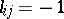oraccording to whetherand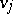are adjacent or not. Given two graphsand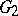onvertices, it is of interest to determine the set of immanantsfor which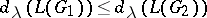. See [a2], [a7], for a characterization of the trees in various families that attain the smallest immanant values. See [a6] for a linear relation between the third hook immanant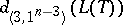of the treeand the chemical index, called the Wiener number of. The relation betweenand other chemical indices is explored in [a4]. The results of Ph. Botti and Merris in [a1] show that immanants of Laplacian matrices of trees do not distinguish non-isomorphic trees in general. More specifically, almost every tree shares the same set of immanant values with another non-isomorphic tree on the same number of vertices.

Linear transformations of a matrix that preserve its immanant values have been investigated in [a8], [a9], [a10]. When an indeterminateis introduced, the immanant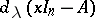may be expanded as a polynomial inof degree. This polynomial is known as the immanantal polynomial of. The immanantal polynomials of graph Laplacians have been studied in [a1], [a29], [a30], [a27], [a45]. Computational issues related to the evaluation of immanants are considered in [a3].